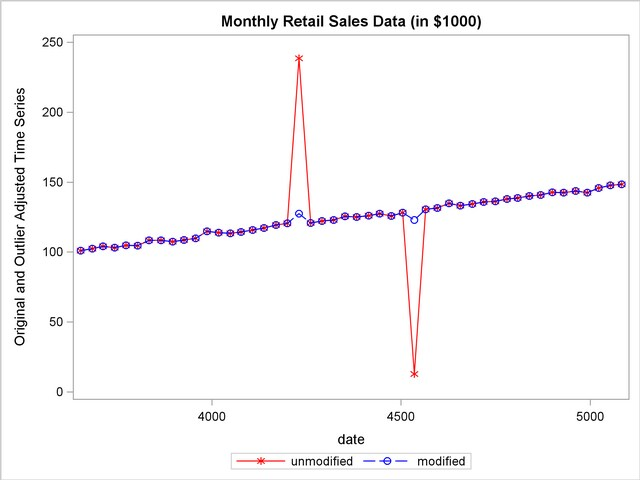The X11 Procedure

## Example 31.3 Outlier Detection and Removal

PROC X11 can be used to detect and replace outliers in the irregular component of a monthly or quarterly series.

The weighting scheme used in measuring the "extremeness" of the irregulars is developed iteratively; thus the statistical properties of the outlier adjustment method are unknown.

In this example, the data are simulated by generating a trend plus a random error. Two periods in the series were made "extreme" by multiplying one generated value by 2.0 and another by 0.10. The additive model is appropriate based on the way the data were generated. Note that the trend in the generated data was modeled automatically by the trend cycle component estimation.

The detection of outliers is accomplished by considering Table D9, the final replacement values for extreme S-I ratios. This table indicates which observations had irregular component values more than FULLWEIGHT= standard deviation units from 0.0 (1.0 for the multiplicative model). The default value of the FULLWEIGHT= option is 1.5; a larger value would result in fewer observations being declared extreme.

In this example, FULLWEIGHT=3.0 is used to isolate the extreme inflated and deflated values generated in the DATA step. The value of ZEROWEIGHT= must be greater than FULLWEIGHT; it is given a value of 3.5.

A plot of the original and modified series, Output 31.3.2, shows that the deviation from the trend line for the modified series is greatly reduced compared with the original series.

```   data a;
retain seed 99831;
do kk = 1 to 48;
x = kk + 100 + rannor( seed );
date = intnx( 'month', '01jan1970'd, kk-1 );
if kk = 20 then x = 2 * x;
else if kk = 30 then x = x / 10;
output;
end;
run;
```
```   proc x11 data=a;
fullweight=3.0 zeroweight=3.5;
var x;
table d9;
output out=b b1=original e1=e1;
run;

proc sgplot data=b;
series x=date y=original / markers
markerattrs=(color=red symbol='asterisk')
lineattrs=(color=red)
legendlabel="unmodified" ;
series x=date y=e1       / markers
markerattrs=(color=blue symbol='circle')
lineattrs=(color=blue)
legendlabel="modified" ;
yaxis label='Original and Outlier Adjusted Time Series';
run;
```

Output 31.3.1 Detection of Extreme Irregulars
 Monthly Retail Sales Data (in \$1000)

The X11 Procedure